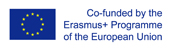This project has been funded with support from the European Commission.
This web site reflects the views only of the author, and the Commission cannot be held responsible for any use which may be made of the information contained therein.

Select language   >   IT EN RO PL LT

### Pythagorean Theorem

Subject taught
- Math

Language Skills Developed
- Interaction
- Listening
- Speaking
- Writing

Transferable/Scientific Skills Developed
Students will develop self-motivation, collaborative learning, problem solving, self-directed and independent learning skills.
Students will be able to prove the Pythagorean Theorem in at least two different ways and to apply it to solving math
problems. Students will learn to apply the theorem in real life situations.

Description
General aims:
-to define the elements of the right triangle in English, define the Pythagorean theorem and to use key vocabulary to prove the Pythagorean theorem.
Linguistic aims
-to introduce key vocabulary: the right angle triange, the square, hypotenuse, legs, side, square corner, tilted, rearrange, rectangles, ratio, equesions,
-to use key vocabulary to prove the Pythagorean theorem,
- to improve listening, reading and speaking skills.
Subject specific aims
Students will be able to:
- define the Pythagorean Theorem,
- prove the Pythagorean Theorem in at least two ways,
- solve equations using the Pythagorean Theorem.
Target group age: lower secondary school students 13 – 15 years old students.
Level of competence in English (CEFR) B1/B1+.
Time required to use the resource with the students: 2-3 lessons.
Having introduced the objectives of the lesson, the teacher introduces key vocabulary.
Students watch the video lesson. The video can be stopped several times to give students some time to think, to ask questions, participate in a discussion.
The teacher asks the students to define the theorem.
To memorise the key vocabulary better, students practice it using created resources.
After watching and analysing the video, students, working individually, apply the Pythagorean theorem to solve mathematical problems.
Working in small groups, students will think of real-life situations in which the Pythagorean theorem could be applied.
The teacher asks the students to reflect on what they have learned in the lesson and summarizes the lesson.
Possible difficulties for the students:
Students who usually find solving mathematical problems difficult may find proving the Pythagorean theorem in English even more difficult.

Teaching Resources (created)

Teaching Resources (reviewed)